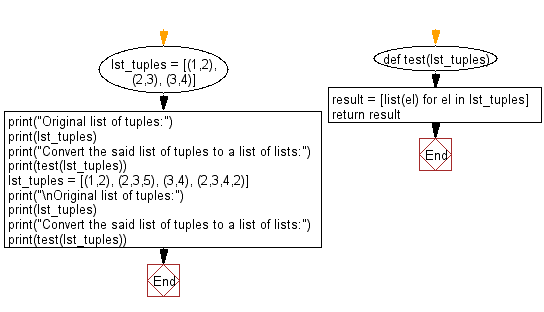﻿ Python: Convert a given list of tuples to a list of lists - w3resource# Python Exercise: Convert a given list of tuples to a list of lists

## Python tuple: Exercise-33 with Solution

Write a Python program to convert a given list of tuples to a list of lists.

Sample Solution:-

Python Code:

``````def test(lst_tuples):
result = [list(el) for el in lst_tuples]
return result
lst_tuples = [(1,2), (2,3), (3,4)]
print("Original list of tuples:")
print(lst_tuples)
print("Convert the said list of tuples to a list of lists:")
print(test(lst_tuples))
lst_tuples = [(1,2), (2,3,5), (3,4), (2,3,4,2)]
print("\nOriginal list of tuples:")
print(lst_tuples)
print("Convert the said list of tuples to a list of lists:")
print(test(lst_tuples))
```
```

Sample Output:

```Original list of tuples:
[(1, 2), (2, 3), (3, 4)]
Convert the said list of tuples to a list of lists:
[[1, 2], [2, 3], [3, 4]]

Original list of tuples:
[(1, 2), (2, 3, 5), (3, 4), (2, 3, 4, 2)]
Convert the said list of tuples to a list of lists:
[[1, 2], [2, 3, 5], [3, 4], [2, 3, 4, 2]]
```

Flowchart:## Visualize Python code execution:

The following tool visualize what the computer is doing step-by-step as it executes the said program:

Python Code Editor:

Have another way to solve this solution? Contribute your code (and comments) through Disqus.

What is the difficulty level of this exercise?

Test your Programming skills with w3resource's quiz.

﻿

## Python: Tips of the Day

Decapitalizes the first letter of a string:

Example:

```def tips_decapitalize(s, upper_rest=False):
return s[:1].lower() + (s[1:].upper() if upper_rest else s[1:])
print(tips_decapitalize('PythonTips'))
print(tips_decapitalize('PythonTips', True))
```

Output:

```pythonTips
pYTHONTIPS
```